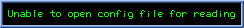Simple Prime Number Generator

Introduction: Here are some programs that I wrote a long time ago that generate prime numbers from a starting point. The algorythm is simple: Test divisibility of every odd number by every odd number from 3 to the last odd number before the square root of the number to be tested. It is a relatively fast way of generating prime numbers without using a sieve.

Note that the programs in the gmpprimes directory use the GNU precision library. On today's processors it is easy to verify 10-digit prime numbers, so in order to verify bigger primes I rewrote my original primes program to use the GNU precision library to verify as big primes as you want!

Just for fun, here is a list of all the prime numbers from one to a million.

charlton@dynet.com

This page has been hittimes since March 1, 2001.